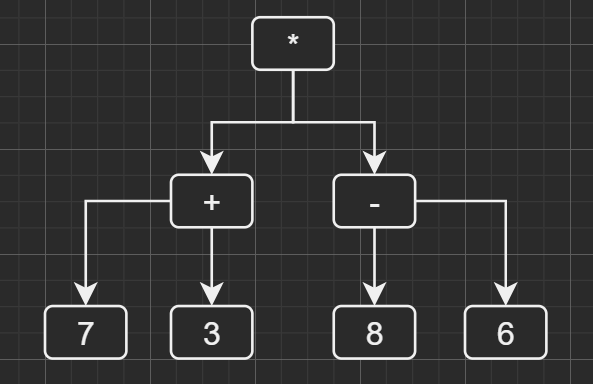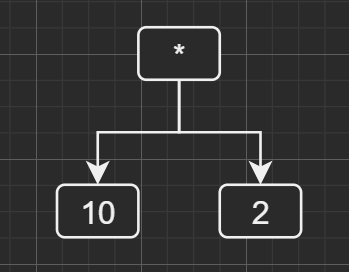• ————Python模运算个人总结归纳———— a和b同为正整数的时候 如果 a < b 则结果为 a 3 % 5 = 3 10 % 20 = 10 123 % 756 = 123 .....诸如此类 如果 a > b 结果为 a — （b在...
————Python模运算个人总结归纳————
a和b同为正整数的时候
如果 a < b 则结果为 a
						 3 % 5 = 3
10 % 20 = 10
123 % 756 = 123
.....诸如此类

如果 a > b 结果为 a — （b在范围a以内的最大倍数值）做差。
						a = 6
b = 2
6 - 2 * 3（6以内2的最大倍数值） = 0
6 % 2 = 0

a = 121
b = 6
121 - 6 * 20（121以内的6的最大倍数值为120）
121 % 6 = 1

a和b同为负整数的时候
如果 a > b 则结果仍为 a
						-4 % -8 = -4
-7 % -9 = -7
-1234 % -5678 = -1234
......诸如此类

如果 a < b 则结果为 a — （b在范围a以内的最大倍数值）做差
						 a = -8
b = -3
-8 - (-3 * 2) (-8 以内-3的最大倍数值为-6） =  -2
-8 % -3 = -2

结论一 同为负的模运算可以遵照同为正的整数模运算在结果前加（—）负号
						-a % -b = -c 可以看作 a % b 后在结果前加符号
-a % -b = -c   等价 a % b = c ,  c取反 = -c

-8 % -3 等价 8 % 3 结果取反
-8 % -3 = -2
8 % 3 = 2 结果取反为 -2

优点一 更加直观不容易出错
a 或者 b 一方为负数时
按照模运算公式
	模运算公式 a % b 等价 a - (b * (a / / b) )

更为直观的判断
无论是 -a % b 还是 a % -b 对我来说都是做和运算
我的运算公式 a%b一方为负时:
b恒取绝对值比a大的最小倍数值做和
当 a < 0 , b > 0的时候结果恒为正 a = -8 b = 3 -8 % 3 = 1 等价 -8 + （3 * 3）“b取绝对值刚好比a大的倍数值” b=3 绝对值刚好比a大的是9 然后两者做和运算 -8 + 9 = 1
					-16 % 12 = 8  等价-16 + 24	= 8
-27 % 13= 12 等价 -27 + 39 =12

当 a > 0 , b < 0的时候结果恒为负 a = 8 b = 3 8 % -3 = -1 等价 -8 + （3 * 3）“b取绝对值刚好比a大的倍数值” b=3 绝对值刚好比a大的是9 然后两者做和运算 8 + -9= -1
					16 % -12 = -8 等价16 + -24 = -8
27 % -13 = -12 等价 27 + -39 = -12

感谢您的阅读，如果能帮到你我和开心！
展开全文• python 二叉树-解析四则运算公式 文章目录python 二叉树-解析四则运算公式1、解析树完全括号表达式2、解析四则运算-Coding 1、解析树 根据括号构建解析数 计算解析树中的表达式 将解析树还原为最初的数学表达式 ...
python 二叉树-解析四则运算公式

文章目录
python 二叉树-解析四则运算公式1、解析树完全括号表达式
2、解析四则运算-Coding

1、解析树
根据括号构建解析数计算解析树中的表达式将解析树还原为最初的数学表达式
构建表达式 **((7+3)*(8-6))**的解析树

先算加减，后算乘法 在计算顶层的乘法前， 需要先计算子树中的加法和减法， 计算完之后使用记过替代整颗子树构建解析树规则
如果标记是 (， 为当前节点创建一个子节点， 并下沉至该节点如果当前标记在列表["+", “-”, “/”, “*”], 将当前节点的值设置为当前标记对应的运算符；为当前节点穿件一个右节点并下沉至该节点如果当前值是是数值， 就将当前节点的数值设置为该数值并返回至父节点如果标记是), 则跳到当前节点的父节点
完全括号表达式
对每一对运算符都添加一对括号， 由括号决定运算顺序， 杜绝歧义， 并且不需要记忆运算规则
2、解析四则运算-Coding
BuildParseTree 类：解析并计算公式
# -*- coding: utf-8 -*-
import operator

class BuildParseTree:

def __init__(self, fpexp):
self.fplist = fpexp.split()

def buildParseTree(self):
"""
构建解析树
使用栈来保存父节点
"""
pStack = Stack()
eTree = BinaryTree('')
pStack.push(eTree)
curentTree = eTree
for i in self.fplist:
# 如果标记是 (， 为当前节点创建一个子节点， 并下沉至该节点
if i == "(":

curentTree.insertLeft("")
pStack.push(curentTree)
curentTree = curentTree.getLeftChild()
# 如果当前标记在列表["+", "-", "/", "*"], 将当前节点的值设置为当前标记对应的运算符；为当前节点穿件一个右节点并下沉至该节点
elif i not in "=-*/":
curentTree.setRootVal(eval(i))
parent = pStack.pop()
curentTree = parent
# 如果当前值是是数值， 就将当前节点的数值设置为该数值并返回至父节点
elif i in "=-*/":
curentTree.setRootVal(eval(i))
curentTree.insertRight("")
pStack.push(curentTree)
curentTree.getRightChild()
# 如果标记是), 则跳到当前节点的父节点
elif i == ")":
parent = pStack.pop()
curentTree = parent
else:
raise ValueError("Error: %s" % i)
return eTree

def evaluate(self, parseTree):
"""
计算解析树
"""

# 构建运算操作字典
opers = {
"-": operator.sub,
"*": operator.mul,
"/": operator.truediv
}

leftC = parseTree.getLeftChild()
rightC = parseTree.getRightChild()
if leftC and rightC:
fn = opers[parseTree.getRootVal()]
return fn(self.evaluate(leftC), self.evaluate(rightC))
else:
return parseTree.getRootVal()

if __name__ == "__main__":
formulas = "((7+3)*(8-6))"
fTree = BuildParseTree(formulas)
eTree = fTree.buildParseTree()
value = fTree.evaluate(eTree)
print("%s = " % formulas, value)

BinaryTree类
# -*- coding: utf-8 -*-

class BinaryTree:
"""
利用节点与引用创建二叉树
"""

def __init__(self, rootObj):
self.key = rootObj
self.leftChild = None
self.rightChild = None

def insertLeft(self, newNode):
"""
插入左节点
"""
if self.leftChild is None:
self.leftChild = BinaryTree(newNode)
else:
t = BinaryTree(newNode)
t.leftChild = self.leftChild
self.leftChild = t

def insertRight(self, newNode):
"""
插入右节点
"""
if self.rightChild is None:
self.rightChild = BinaryTree(newNode)
else:
t = BinaryTree(newNode)
t.rightChild = self.rightChild
self.rightChild = t

def getRightChild(self):
"""
返回当前节点的右节点和对应的二叉树
"""
return self.rightChild

def getLeftChild(self):
"""
返回当前节点的左节点和对应的二叉树
"""
return self.leftChild

def getRootVal(self):
"""
获取当前节点的数值
"""
return self.key

def setRootVal(self, obj):
"""
存储当前节点的数值
"""
self.key = obj


Stack 类
# -*- coding: utf-8 -*-

class Stack():

def __init__(self):
self.items = list()

def isEmpty(self):
return self.items == []

def push(self, item):
self.items.append(item)

def pop(self):
return self.items.pop()

def peek(self):
return self.items[-1]

def size(self):
return len(self.items)

if __name__ == "__main__":
stack = Stack()
stack.push(1)
stack.push(2)
stack.push(3)
stack.push(4)
print(stack.items)
print(stack.peek())
print(stack.isEmpty())

展开全文二叉树
• python 栈-解析四则运算公式 文章目录python 栈-解析四则运算公式1、栈栈的抽象数据类型解析算数表达式中序转后序后序表达式计算2、解析四则运算-Coding 1、栈 栈也被称为下堆栈， 他是有序集合， 添加操作和移除...
python 栈-解析四则运算公式

文章目录
python 栈-解析四则运算公式1、栈栈的抽象数据类型解析算数表达式中序转后序后序表达式计算
2、解析四则运算-Coding

1、栈
栈也被称为下堆栈， 他是有序集合， 添加操作和移除操作发生在同一端，即顶端， 另一端称为底端
LIFO(last-in, first-out) 后进先出可用于反转排列顺序
栈的抽象数据类型
支持以下操作
push(item) 将元素添加到栈中pop() 返回并删除顶端的元素peek() 返回顶端的元素， 但不删除元素isEmpty() 判断栈是否为空size() 返回栈中的元素数量
解析算数表达式
括号匹配： 每一个左括号都有与之对应的又括号， 并且括号有正确的嵌套关系

处理括号匹配 由空栈开始，从左往右依次处理括号。遇到左括号， 使用push操作将其加入栈中；如果遇到右括号就调用pop操作, 所有的左括号都应有右括号与之匹配。 处理完括号匹配，栈应该是空的

前序表达式：运算符调整到两个操作数前, eg: +ab  中序表达式：运算符出现在两个操作数间, eg: a*b  后序表达式：运算符调整至操作数后, eg: ab+  完全括号表达式: 对每一对运算符都添加一对括号， 由括号决定运算顺序， 杜绝歧义， 并且不需要记忆运算规则
中序表达式前序表达式后序表达式a + b+ a ba b +a - b / c- a / b ca b c / -a + b * c + d+ a * b c + da b c * + d +(a + b) * ( c + d)* + a b + c da b + c d + *
中序转后序
创建一个空栈和列表， 分别保存运算符和转换结果列表将中序表达式转换为一个列表从左往右扫描列表
数值， 添加至转换列表中左括号， 压入栈中右括号， 重复从栈中获取元素，添加至转换结果列表中， 直至获取左括号运算符，将其与栈中的顶端元素比较，从栈中取出优先级高的元素追加至转换列表中 处理完表达式后将栈中残余的运算符追加至转换结果列表中
后序表达式计算
创建空栈将后续表达式转化为一个列表从左往右扫描列表
数值，压入栈中运算符，从栈中去两个元素， 第一个为右数， 第二个为左数，计算出数值后重新压入栈中 处理完表达式后， 从栈中获取数值
2、解析四则运算-Coding
后序表达式计算
# -*- coding: utf-8 -*-

"""
中序表达式转后序表达式, 并计算
"""
import re
import operator
import string

class PostOrderConversion:

def conversionToPostOrder(self, inOrdErexpr):
"""
后序转换
"""
# 构建运算符优先级字典
prec = {
"*": 3,
"/": 3,
"+": 2,
"-": 2,
"(": 1,
")": 1
}

if not self.parseChecker(inOrdErexpr):
raise ValueError

opStack = Stack()
postOrderList = list()
exprList = inOrdErexpr.split()

for ch in exprList:
if ch.isdigit():
postOrderList.append(ch)
elif ch == "(":
opStack.push(ch)
elif ch == ")":
topOper = opStack.pop()
while topOper != "(":
postOrderList.append(topOper)
topOper = opStack.pop()
else:
# 比较运算符优先级，如果栈中运算符的优先级>当前运算符， 追加至转换列表中
while (not opStack.isEmpty()) and (prec[opStack.peek()] > prec[ch]):
postOrderList.append(opStack.pop())
opStack.push(ch)
# 将栈中的运算符追加至转换列表中
while not opStack.isEmpty():
postOrderList.append(opStack.pop())
return "".join(postOrderList)

def calculatePostOrderFormulas(self, postOrderformulas):
"""
计算后序表达式
"""
"-": operator.sub,
"*": operator.mul,
"/": operator.truediv
}
postStack = Stack()
postOrderList = list(postOrderformulas)
for ch in postOrderList:
if ch.isdigit():
postStack.push(eval(ch))
else:
opN1 = postStack.pop()
opN2 = postStack.pop()
return postStack.pop()

def parseChecker(self, symbolStr):
"""
判断括号是否完全匹配
"""
symbolStr = self.extractBrackets(symbolStr)
s = Stack()
balanced = True
index = 0
while index < len(symbolStr) and balanced:
symbol = symbolStr[index]
if symbol in "{[(":
s.push(symbol)
elif s.isEmpty():
balanced = False
else:
topSym = s.pop()
if self.matches(topSym, symbol):
balanced = False
index += 1
if balanced and s.isEmpty():
return True
else:
return False

def extractBrackets(self, formulas):
regex = re.compile(r'[{$()$}]')
return "".join(regex.findall(formulas))

def matches(self, open, close):
opens = "{[("
closers = "}])"
return opens.index(opens) == closers.index(close)

if __name__ == "__main__":
formulas1 = "( 1 + 2 ) * 3"
formulas2 = "5 + 6 * 7"
postConversion = PostOrderConversion()
cFormulas1 = postConversion.conversionToPostOrder(formulas1)
cFormulas2 = postConversion.conversionToPostOrder(formulas2)
print("formulas1: %s | formulas1: %s" % (cFormulas1, cFormulas1))
print("formulas1: %s=" % formulas1, postConversion.calculatePostOrderFormulas(cFormulas1))
print("formulas2: %s=" % formulas2, postConversion.calculatePostOrderFormulas(cFormulas2))


计算结果
formulas1: 12+3* | formulas1: 12+3*
formulas1: ( 1 + 2 ) * 3= 9
formulas2: 5 + 6 * 7= 47

创建栈
# -*- coding: utf-8 -*-

class Stack():

def __init__(self):
self.items = list()

def isEmpty(self):
return self.items == []

def push(self, item):
self.items.append(item)

def pop(self):
return self.items.pop()

def peek(self):
return self.items[-1]

def size(self):
return len(self.items)

if __name__ == "__main__":
stack = Stack()
stack.push(1)
stack.push(2)
stack.push(3)
stack.push(4)
print(stack.items)
print(stack.peek())
print(stack.isEmpty())

展开全文算法
• 1 abs(x) #返回绝对值 2 x+y,x-y,x*y,x/y #加减乘除 3 x/y #取商，浮点数相除保留余数 4 x//y #取商，浮点数相除余数为0 5 x%y #取余 6 x**y #幂次方 7 divmod(x,y) ...

1 abs(x)      　　  #返回绝对值
2 x+y,x-y,x*y,x/y  #加减乘除  3 x/y #取商，浮点数相除保留余数  4 x//y #取商，浮点数相除余数为0  5 x%y #取余  6 x**y #幂次方   7 divmod(x,y) #相除得到商和余数组成的元组  8 float(x) 　　　#转换为浮点型  9 str(x) 　　 #转换为字符串 10 hex(x) 　　 #转换为16进制 11 oct(x) 　　 #转换8进制

转载于:https://www.cnblogs.com/anchao88/p/8709551.html
展开全文• Python近几年来越来越受到各行各业的喜爱，主要还是因为Python语言的简洁性，下边小编就带大家一起来看一下用Python来实现我们小学时候的加减乘除运算时有多么的简单！实例：加减乘除运算我们先来看一下代码，然后再...
• ## python数组运算

千次阅读 2017-10-19 21:15:31
本文摘自《用Python做科学计算》，版权归原作者所有。 1. NumPy-快速处理数据--ndarray对象--数组的创建和存取 2. NumPy-快速处理数据--ndarray对象--多维数组的存取、结构体数组存取、内存对齐、Numpy内存结构 ...数组 矩阵
• 做了一些 利用python进行数值间的双目运算、取整、复数运算、求导、求倒数运算的示例代码
• ## Python 符号运算

千次阅读 2020-12-12 14:57:53
背景介绍： Sugar 起初在 MultiMCU EDU 上写的都是有飞控、嵌入式操作系统、控制算法类的推文。 最近推的 Matlab 和 Python 似乎与 MCU...本篇 Sugar 就来说一说 Python 中的符号运算。 sympy 一、安装 sympy 模块 Su.
• 区分与其他语言，对于x%y，如果都是整数，则返回x/y的余数；如果是浮点数，返回的是x - int(x/y)*y ;如果是复数，返回的是x - int((x/y).real)*y...不过以上这些公式貌似都只是对于操作数都是整数的情况下才满足。。。
• python的加减乘除： 加：a = b + c 减：a = b - c 乘：a = b * c 除：a = b / c (此处与java不同，这里求出来的为小数，并不是整数位) 求余：a = b % c 次方：a = b ** c (b的c次方)...
• scale = 2 ** -s exp_diag = np.exp(scale * diag_T) for k in range(n): X[k, k] = exp_diag[k] 错误消息X[k, k] = exp_diag[k] TypeError: only length-1 arrays can be converted to Python scalars 向我建议exp...
• Python 取模运算（取余）%误区及详解功能快捷键合理的创建标题，有助于目录的生成如何改变文本的样式插入链接与图片如何插入一段漂亮的代码片生成一个适合你的列表创建一个表格设定内容居中、居左、居右SmartyPants...
• python进行运算时，如果会出现浮点数，有时候会需要对小数精度进行控制，主要方法有以下几种：1、round（）round()是python的内置方法， round()如果只有一个数作为参数，不指定位数的时候，返回的是一个整数，...小数精度 decimal getcontext Decimal
• 前言 从小学我们都知道，三角形的面积是底乘以高除以2。那么已知任意一个三角形的三条边，如何能够求出三角形的...python根据三角形三条边求面积 1.三角形的三条边的符合条件 我们知道，三角形有三条边，且三条边...
• 数学 库，带有使用Python处理数学函数和公式的工具。
• 模运算，用得算是比较多，但是负数的模运算还是用的比较少 -1 % 8 = 7 1 % -8 = -7 -1 % -8 = -1 1 % 8 = 1 从上述的例子可以看出，如果模运算的算术符符号相同，则直接等于取绝对值以后，再做模运算（负数则是负...
• ## python常用数学公式

千次阅读 2019-06-17 16:33:28
4、**求幂运算：5**3=125； 进制转换： print(int("0b100",2))将2进制转10进制 print(int("0o100",8))将8进制转10进制 print(int("0x100",16))将16进制转10进制 print(bin(321)) 10进制转2...
• 机器学习数学基础之 Python 矩阵运算（人工智能与机器学习）一、Python 矩阵基本运算1. Python 矩阵操作2. Python 矩阵乘法3. 矩阵的转置4. Python 求方阵的迹5. Python 方阵的行列式计算方法6. Python 求逆矩阵/...
• 本章从计算数学公式的程序编写开始，学习如何使用 Python 这种计算机语言来编程，编程就是编写程序。本章还要学习如何使用变量，如何计算 和 这样的数学函数，以及如何把 Python 当作计算器一样交互式使用。 编程...
• 题目内容： 对于三角形，三边长分别为a, b, c，给定a和b之间的夹角C，则有：。编写程序，使得输入三角形的边a, b, c，可求得夹角C(角度值)。 输入格式： 三条边a、b、c的长度值，每个值占一行。...
• 给出一段如下行，比较复杂的运算公式，自己编写代码计算 1 - 2.99 * ( (60.2-30 +( -40/ 5) * (9-2*5/3 + 7 /3*99/4*2998 +10.5 * 568/14 )) - (-4*3)/ (16-3*2) ) 思路： step1.需要先检查合法性，检测有无...
• 文章目录一、实验说明二、Python 矩阵基本运算1. python矩阵操作2. python矩阵乘法3. python矩阵转置4. python求方阵的迹5. python方阵的行列式计算方法6. python求逆矩阵/伴随矩阵7. python解多元一次方程 一、实验...numpy
• 今天小编就为大家分享一篇Python中的取模运算方法，具有很好的参考价值，希望对大家有所帮助。一起跟随小编过来看看吧
• python中可以做的数学运算，仅局限在了基本数据类型int、float。因为我们前面学过了特殊方法，所以我们现在也可以进行有理数运算、矩阵运算，今天我们就先讲解有理数运算，矩阵运算与有理数运算方法基本一样，都要...Rational 求最大公约数
• 今天碰到一道有意思的题目，看了之后发现自己对Python与或的理解还是欠缺，如下。 题目：求1+2+…+n 来源：Leetcode 如果不加限制，我们有很多方法计算该值，例如高斯公式，递归等。 我们思考下递归的解法： def ......

# python模运算公式python 订阅